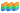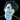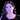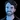# Compact word vectors with Bloom embeddings

### Apr 26, 2022· byMatthew Honnibal, Adriane Boyd & Vincent D. Warmerdam· ~14 min. read

A high-coverage word embedding table will usually be quite large. One million 32-bit floats occupies 4MB of memory, so one million 300-dimension vectors will be 1.2GB in size. Such a large model size is at least annoying for many applications, while for others it’s completely prohibitive.

There are three obvious approaches to reducing the size of the embedding table:

1. Reduce the number of words in the vocabulary.

2. Reduce the number of dimensions per vector.

3. Reduce the number of bits per dimension.

While all three of these options can be effective, there’s also a less obvious solution:

4. Cheat, using a probabilistic data structure.

Probabilistic data structures are a natural fit for machine learning models, so they’re quite widely used. However, they’re definitely unintuitive, which is why we refer to this solution as a “cheat”. We’ll start by introducing the full algorithm, without dwelling too long on why it works. We’ll then go back and fill in more of the intuition, and then describe how we use it in practice in Thinc, spaCy and floret.

## The Bloom embeddings algorithm

In a normal embedding table, each word-string is mapped to a distinct ID. Usually these IDs will be sequential, so if you have a vocabulary of 100 words, your words will be mapped to numbers `range(100)`. The sequential IDs can then be used as indices into an embedding table: if you have 100 words in your vocabulary, you have 100 rows in the table, and each word receives its own vector.

However, there’s no limit to the number of unique words that might occur in a sample of text, while we definitely want a limited number of rows in our embedding table. Some of the rows in our table will therefore need to be shared between multiple words in our vocabulary. One obvious solution is to set aside a single vector in the table. Words 0-98 will each receive their own vector, while all other words are assigned to vector 99.

However, this asks vector 99 to do a lot of work. What if we gave more vectors to the unknown words?

Instead of only one unknown word vector, we could have 10: words 0–89 would be assigned their own vector as before. However, all other words would be randomly mapped to the remaining 10 vectors:

``````
def get_row(word_id, number_vector=100, number_oov=10):
number_known = number_vector - number_oov
if word_id < number_known:
return word_id
else:
return number_known + (word_id % number_oov)
``````

This gives the model a little more resolution for the unknown words. If all out-of-vocabulary words are assigned the same vector, then they’ll all look identical to the model. Even if the training data actually includes information that shows two different out-of-vocabulary words have important, different implications – for instance, if one word is a strong indicator of positive sentiment, while the other is a strong indicator of negative sentiment – the model won’t be able to tell them apart. However, if we have 10 buckets for the unknown words, we might get lucky, and assign these words to different buckets. If so, the model would be able to learn that one of the unknown-word vectors makes positive sentiment more likely, while the other vector makes negative sentiment more likely.

If this is good, then why not do more of it? Bloom embeddings are like an extreme version, where every word is handled like the unknown words above: there are 100 vectors for the “unknown” portion, and 0 for the “known” portion.

So far, this approach seems weird, but not necessarily good. The part that makes it unfairly effective is the next step: by simply doing the same thing multiple times, we can greatly improve the resolution, and have unique representations for far more words than we have vectors. The code in full:

``````import numpy
import mmh3

def allocate(n_vectors, n_dimensions):
table = numpy.zeros((n_vectors, n_dimensions), dtype='f')
table += numpy.random.uniform(-0.1, 0.1, table.size).reshape(table.shape)
return table

def get_vector(table, word):
hash1 = mmh3.hash(word, seed=0)
hash2 = mmh3.hash(word, seed=1)
row1 = hash1 % table.shape
row2 = hash2 % table.shape
return table[row1] + table[row2]

def update_vector(table, word, d_vector):
hash1 = mmh3.hash(word, seed=0)
hash2 = mmh3.hash(word, seed=1)
row1 = hash1 % table.shape
row2 = hash2 % table.shape
table[row1] -= 0.001 * d_vector
table[row2] -= 0.001 * d_vector
``````

In this example, we’ve used two keys, assigned from two random hash functions. It’s unlikely that two words will collide on both keys, so by simply summing the vectors together, we’ll assign most words a unique representation.

For the sake of illustration, let’s step through a very small example, explicitly.

Let’s say we have this vocabulary of 20 words:

``````vocab = ['apple', 'strawberry', 'orange', 'juice',
'drink', 'smoothie', 'eat', 'fruit',
'health', 'wellness', 'steak', 'fries',
'ketchup', 'burger', 'chips', 'lobster',
'caviar', 'service', 'waiter', 'chef']
``````

We’ll embed these into two dimensions. Normally this would give us a table of `(20, 2)` floats, which we would randomly initialise. With the hashing trick, we can make the table smaller. Let’s give it 15 vectors:

``````normal = numpy.random.uniform(-0.1, 0.1, (20, 2))
hashed = numpy.random.uniform(-0.1, 0.1, (15, 2))
``````

In the normal table, we want to map each word in our vocabulary to its own vector:

``````word2id = {}
def get_normal_vector(word, table):
if word not in word2id:
word2id[word] = len(word2id)
return normal[word2id[word]]
``````

The hashed table only has 15 rows, so some words will have to share. We’ll handle this by mapping the word into an arbitrary integer – called a “hash value”. The hash function will return an arbitrary integer, which we’ll mod into the range `(0, 15)`. Importantly, we need to be able to compute multiple, distinct hash values for each key – so Python’s built-in hash function is inconvenient. We’ll therefore use MurmurHash.

Let’s see what keys we get for our 20 vocabulary items, using MurmurHash:

``````hashes1 = [mmh3.hash(w, 1) % 15 for w in vocab]
assert hashes1 == [3, 6, 4, 13, 8, 3, 13, 1, 9, 12, 11, 4, 2, 13, 5, 10, 0, 2, 10, 13]
``````

As you can see, some keys are shared between multiple words, while 2/15 keys are unoccupied. This is obviously unideal! If multiple words have the same key, they’ll map to the same vector – as far as the model is concerned, “strawberry” and “heart” will be indistinguishable. It won’t be clear which word was used – they have the same representation.

To address this, we simply hash the words again, this time using a different seed – so that we get a different set of arbitrary keys:

``````from collections import Counter

hashes2 = [mmh3.hash(w, 2) % 15 for w in vocab]
assert len(Counter(hashes2).most_common()) == 12
``````

This one’s even worse – 3 keys unoccupied! But our strategy is not to keep drawing until we get a favorable seed. Instead, consider this:

``````assert len(Counter(zip(hashes1, hashes2))) == 20
``````

By combining the results from the two hashes, our 20 words distribute perfectly, into 20 unique combinations. This makes sense: we expect to have some words overlapping on one of the keys, but we’d have to be very unlucky for a pair of words to overlap on both keys.

This means that if we simply add the two vectors together, each word once more has a unique representation:

``````for word in vocab:
key1 = mmh3.hash(word, 0) % 15
key2 = mmh3.hash(word, 1) % 15
vector = hashed[key1] + hashed[key2]
print(word, '%.3f %.3f' % tuple(vector))
``````
``````apple 0.161 0.163
strawberry 0.128 -0.024
orange 0.157 -0.047
juice -0.017 -0.023
drink 0.097 -0.124
smoothie 0.085 0.024
eat 0.000 -0.105
fruit -0.060 -0.053
health 0.166 0.103
wellness 0.011 0.065
steak 0.155 -0.039
fries 0.157 -0.106
ketchup 0.076 0.127
burger 0.045 -0.084
chips 0.082 -0.037
lobster 0.138 0.067
caviar 0.066 0.098
service -0.017 -0.023
waiter 0.039 0.001
chef -0.016 0.030
``````

We now have a function that maps our 20 words to 20 unique vectors – but we’re storing weights for only 15 vectors in memory. Now the question is: will we be able to find values for these weights that let us actually map words to useful vectors?

Let’s do a quick experiment to see how this works. We’ll assign “true” values for our little vocabulary, and see how well we can approximate them with our compressed table. To get the “true” values, we could put the “science” in data science, and drag the words around into reasonable-looking clusters. But for our purposes, the actual “true” values don’t matter. We’ll therefore just do a simulation: we’ll assign random vectors as the “true” state, and see if we can learn values for the hash embeddings that match them.

The learning procedure will be a simple stochastic gradient descent:

``````import numpy
import mmh3

numpy.random.seed(0)

nb_epoch = 20
learn_rate = 0.001
nr_hash_vector = 15

words = [str(i) for i in range(20)]
true_vectors = numpy.random.uniform(-0.1, 0.1, (len(words), 2))
hash_vectors = numpy.random.uniform(-0.1, 0.1, (nr_hash_vector, 2))
examples = list(zip(words, true_vectors))

for epoch in range(nb_epoch):
numpy.random.shuffle(examples)
loss = 0.0
for word, truth in examples:
key1 = mmh3.hash(word, 0) % nr_hash_vector
key2 = mmh3.hash(word, 1) % nr_hash_vector

hash_vector = hash_vectors[key1] + hash_vectors[key2]

diff = hash_vector - truth

hash_vectors[key1] -= learn_rate * diff
hash_vectors[key2] -= learn_rate * diff
loss += (diff**2).sum()
print(epoch, loss)
``````

It’s worth taking some time to play with this simulation. You can start by doing some sanity checks:

• How does the loss change with `nr_hash_vector`?
• If you remove `key2`, does the loss go up?
• What happens if you add more hash keys?
• What happens as the vocabulary size increases?
• What happens when more dimensions are added?
• How sensitive are the hash embeddings to the initial conditions? If we change the random seed, do we ever get unlucky?

If you play with the simulation for a while, you’ll start to get a good feel for the dynamics, and hopefully you’ll have a clear idea of why the technique works.

## HashEmbed: Bloom embeddings in Thinc and spaCy

spaCy’s `MultiHashEmbed` and `HashEmbedCNN` use the `HashEmbed` layer from Thinc to construct small vector tables with Bloom embeddings for spaCy’s CNN pipelines like `en_core_web_sm`.

`HashEmbed` uses MurmurHash to hash a 64-bit key, which is typically a value from the `StringStore`, to four rows in a small hash table. The final embedding is the sum of the four rows from the table.

If there are multiple attributes such as `[ORTH, PREFIX, SUFFIX, SHAPE]`, `MultiHashEmbed` uses a different seed for each attribute and then concatenates the vectors for each individual attribute to create the context-independent `Tok2Vec` embeddings.

As an example, let’s take a look at the config for `MultiHashEmbed`:

``````[components.tok2vec.model.embed]
@architectures = "spacy.MultiHashEmbed.v2"
width = 96
attrs = ["ORTH","PREFIX","SUFFIX","SHAPE"]
rows = [5000,2500,2500,2500]
``````

There are four attributes with 5000 rows for representing `ORTH`, 2500 for `PREFIX`, etc. The entire embedding table contains 12,500 96-dimension vectors, which takes up less than 5MB.

And in terms of the model size, there’s yet another advantage to relying on hash functions to map strings to rows: given the same hash function, the same string always maps to the same hash, so the model does not even need to store a list of known vocab items like it would for a traditional sequentially-numbered vocab. Any arbitrary string can be mapped into this table, and the model does not require a stored vocab.

## floret: Bloom embeddings for fastText

floret is an extended version of fastText that uses Bloom embeddings to create compact vector tables with both word and subword information.

fastText uses character n-gram subwords to be able to provide vectors for any possible word. A word’s vector is the average of the vector for the full word (if available) and the vectors for all of its subwords. For example, the vector for `apple` with 4-gram subwords would be the average of the vectors for the following strings (`<` and `>` are added as word boundary characters):

``````<apple>
<app
appl
pple
ple>
``````

fastText also supports a range of n-gram sizes, so with 4-grams through 6-grams, you’d have:

``````<apple>
<app
appl
pple
ple>
<appl
apple
pple>
<apple
apple>
``````

By using subwords, fastText models can provide useful vectors for previously unseen tokens like `appletrees` by using subwords like `<appl` and `tree`. Instead of having a single `UNK`-vector, fastText models with subwords can produce better representations for infrequent, novel or noisy words.

Internally fastText stores the word and subword vectors in two separate tables. The word table contains a fixed vocab of words corresponding to tokens above a minimum count in the training data, typically 1–2M words. For subwords, fastText runs into the same issue as we did for our original vector example: it wants to store vectors for a very large number of subwords in a fixed-sized table. fastText uses the same idea with using the full table for the “unknown” portion and hashes each subword into a large table. Since there are typically a very large number of possible subwords (for just 26 lowercase letters there are nearly 12M possible 5-grams), the default hash table size is relatively large at 2M rows. (As a side note, the huge subword table is why fastText `.bin` files are so large even for small vocabs.)

The exported `.vec` table that you can import into a spaCy pipeline only includes the known words, so while the fastText training takes subwords into account, the spaCy pipeline vectors still end up restricted to a fixed list of known vocab words. spaCy could potentially support both the word and subword tables, however with 2M word vectors and 2M subword vectors with 300 dimensions, you’re looking at 4GB+ data, which is definitely prohibitive in many cases.

Here is where Bloom embeddings come into play: with the hashing trick, it’s possible to both greatly reduce the size of the vector table and also support subwords. We can have performant vectors with subword support in under 100MB with just two small changes to fastText:

• store both word and subword vectors in the same hash table
• hash each entry into more than one row

floret extends fastText to implement these two options. In floret mode, the hashing algorithm switches from fastText’s default hash function to MurmurHash for easy integration with Thinc and spaCy. Because each token can be broken down into a large number of subwords, using four hashes per entry as in `HashEmbed` would mean that a long word might correspond to 100+ rows in the table, which can become slow and unwieldy. In practice, just two hashes perform well in medium-sized tables (50–200K) and it’s faster both in training and inference.

With subword support in spaCy vectors, you can see improvements for misspellings and noisy data, for long compounds in languages like German, and across the board for agglutinative languages like Finnish, Korean or Turkish. Even with a floret vector table with 50K entries, which is trained on a relatively small amount of text, you can see large improvements in performance.

You can try out floret in several demo projects:

Coming soon: exploring the advantages of floret for noisy data, novel words, rich morphology and more!

•••